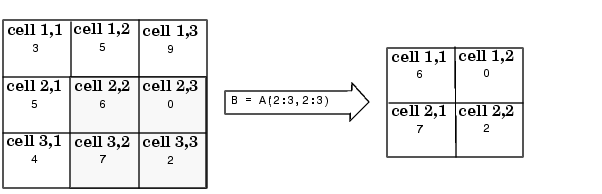ProgrammingObtaining Data from Cell Arrays

You can obtain data from cell arrays and store the result as either a standard array or a new cell array. This section covers

• Accessing cell contents using content indexing
• Accessing a subset of cells using cell indexing

Accessing Cell Contents Using Content Indexing

You can use content indexing on the right side of an assignment to access some or all of the data in a single cell. Specify the variable to receive the cell contents on the left side of the assignment. Enclose the cell index expression on the right side of the assignment in curly braces. This indicates that you are assigning cell contents, not the cells themselves.

Consider the 2-by-2 cell array `N`:

• ```N{1,1} = [1 2; 4 5];
N{1,2} = 'Name';
N{2,1} = 2-4i;
N{2,2} = 7;
```

You can obtain the string in `N{1,2}` using

• ```c = N{1,2}
c =
Name
```

 Note    In assignments, you can use content indexing to access only a single cell, not a subset of cells. For example, the statements `A{1,:} = ``value` and `B = A{1,:}` are both invalid. However, you can use a subset of cells any place you would normally use a comma-separated list of variables (for example, as function inputs or when building an array). See Replacing Lists of Variables with Cell Arrays for details.

To obtain subsets of a cell's contents, concatenate indexing expressions. For example, to obtain element `(2,2)` of the array in cell `N{1,1}`, use

• ```d = N{1,1}(2,2)
d =
5
```

Accessing a Subset of Cells Using Cell Indexing

Use cell indexing to assign any set of cells to another variable, creating a new cell array. Use the colon operator to access subsets of cells within a cell array.Creating Cell Arrays Deleting Cells© 1994-2005 The MathWorks, Inc.